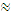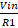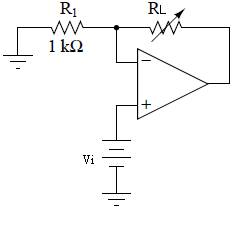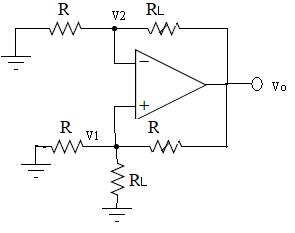Experiment No:05

Title: To design, build and test V-I converter for given specifications. Grounded and floating load.

AIM : To design, build and test V-I converter for given specifications. Grounded and floating load.

APPARATUS REQUIRED: OPA544 Power Op-Amp, INA111 Instrumentation Amplifier, LF411 Op-amp, resistors, Thermistor.

THEORY: Voltage to current converter

A voltage to current converter accepts the input in the voltage form (V i) & produces output in the form of current (I0 ).The output current is proportional to input voltage.

. . . I0=AVi

Where A is called as the sensitivity of V to I converter, in Ampere per volt. The voltage to current (V to I) converters can be classified into two categories, depending on the position of the load.

They are:

1 V to I converter with floating load.

2 V to I converter with grounded load.

Voltage to current converter with floating load:

fig shows a voltage to current converter with floating load. This load (RL) is called as floating load because it is not connected to ground.

Operation:

The input voltage is applied to the non inverting (+) terminal of the OP-AMP. Load resistance RL is connected in place of the feedback resister RF (in the conventional non inverting amplifier).

This circuit is also called as current series negative feedback amplifier. This is because the feedback voltage across R1 is proportional to the output current Io & appears in series with the input voltage.

Apply KVL to the input loop of fig to write,

Vin=Vd+VF

Assu me that the OP-AMP is an ideal one. Hence Vd0

.âˆ™ . Vin =VF

But Vin=R1 Ã— IO (as IB = 0)

. â€˜ . IO =Sensitivity of this circuit is (1/R1). Hence sensitivity can be increased by decreasing the value of R1. Voltage to current converter is used in applications such as low voltage dc &ac voltmeters, LED & zener diode testers etc.

Voltage to current converter with grounded Load:

This is the other type of V to I converter, in which one terminal of the load is connected to the ground.

Circuit Diagram:Fig: Voltage to current converter with floating loadFig: Voltage to current converter with grounded Load

###### PROCEDURE:
1. Design the circuit & connect it as shown in ckt diagram.

2. Apply the Vin & measure the current theoretically & practically.

3. Repeat the above procedure to take the different readings.

4. Note observation & plot the graph.

OBSERVATION TABLE:

Voltage to current converter with floating load

 Sr.No. Vin Ith Ipr

Voltage to current converter with grounded Load

 Sr. No. Vin Ith Ipr

###### Conclusion:

------------------------------------------------------------------------------------------------------------------------------------------------------------------------------------------------------------------------------------------------------------------------------------------------------------------------------------------------------------